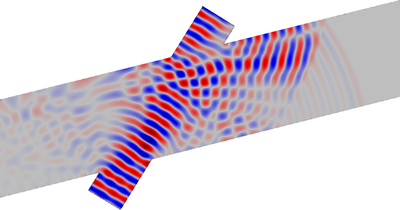# Bibliothèque d'Applications

#### Ultrasound Flowmeter with Generic Time-of-Flight Configuration

Application ID: 34991

Knowing the velocity of a moving fluid is important in all cases where the fluid is used to transport material or energy. In the time-of-flight or transit-time method for determining flow velocity, an ultrasonic signal is transmitted across the main flow in a pipe to noninvasively determine its velocity. By transmitting the signal at an angle relative to the main flow, the ultrasound signal will travel faster than the speed of sound if it moves in the direction of the main flow, and slower than the speed of sound if it moves against it. The difference in travel times in the two directions increases linearly with the velocity of the main flow. Flowmeters of this type find many uses, particularly in industrial settings.

In this tutorial model, learn how to simulate a generic wetted transient-time ultrasound flowmeter with COMSOL Multiphysics® simulation software. The model setup solves the transient problem of a signal traversing the flow downstream. First, we use the CFD Module to calculate the steady-state background flow in the flowmeter. The signal moving upstream is precalculated and imported as data. The difference in arrival times is used to estimate the velocity of the main flow. Next, we use the Convected Wave Equation, Time Explicit physics interface, found under the Ultrasound node in the Acoustics Module. This interface is tailored for transient high-frequency situations and is based on the discontinuous Galerkin method (DG-FEM).This model example illustrates applications of this type that would nominally be built using the following products: